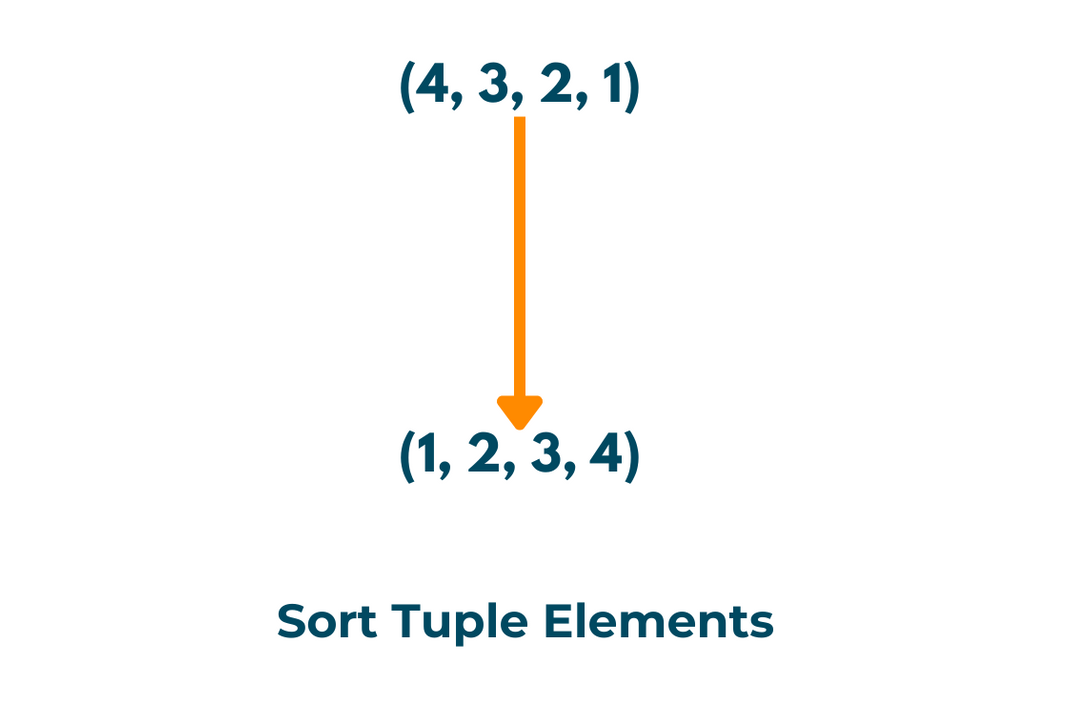# Sort Tuple in Python: Ascending & Decreasing Order (with code)

Tuples were first introduced in python, and they have since been implemented in C++, Java, and C#. They have the primary advantage of allowing developers to store multiple values in a single, compact data structure. In this article, we will learn how to sort tuples in python with code.

## What are Tuples?

A tuple is a data structure that represents an ordered, immutable collection of elements. Its elements can be of different data types and any size.

As mentioned above, one big advantage of tuples is that they are immutable. It means that once a tuple is generated, its values cannot be modified. This makes them ideal for recording data that does not change over time, such as a person's name and birth date. Developers can ensure that the data stored in tuples remain consistent throughout the execution of a program.

Tuples are defined in Python using parentheses and comma-separated values, as shown below:

```individual = ("Abrar Ahmed", 20, "Male")
```

Tuples are defined in a different way in other programming languages, like using square brackets or angle brackets. The core concept of tuples is the same regardless of the syntax used to describe them.

## How to sort Tuples in Python?

Sorting allows us to arrange the tuples in a specific order, such as ascending or descending order, which can make data analysis and processing easier. It can also improve the efficiency of searching for elements in tuples. One more reason to sort is to compare the values in tuples and make better judgments.There are various ways for sorting tuples in python. We will discuss a few of them below:

### 1) Sort method in Ascending Order

In Python, the sort function is used to sort lists in ascending or descending order. This approach, however, can also be used to sort tuples, but some additional steps are required.

To use the sort method on a tuple, first, convert it to a list and then use the sort function on the list. If necessary, the sorted list can be transformed back into a tuple.

Here's an example:

```numbers = (4, 1, 3, 2)

numbers = list(numbers)

numbers.sort()

numbers = tuple(numbers)

print(numbers)
```

Output:

`(1, 2, 3, 4)`

### 2) Sort Method in Descending Order

It should be noted that the sort method can also take an optional argument reverse, which is a Boolean value indicating whether the list should be sorted ascending or descending. If the reverse option is enabled, the list will be arranged in descending order. As an example:

```numbers = (4, 1, 3, 2)

numbers = list(numbers)

numbers.sort(reverse=True)

numbers = tuple(numbers)

print(numbers)
```

Output:

``` (4, 3, 2, 1)
```

### 3) Sorted method

In Python, the sorted method sorts an iterable (such as a list, tuple, or dictionary) in ascending or descending order and produces a new sorted list. The sorted method, unlike the sort method, does not modify the original iterable and instead returns a new iterable.

Here's an example of how to use the sorted method to sort a tuple of numbers in ascending order:

```numbers = (4, 1, 3, 2)

sorted_numbers = sorted(numbers)

print(sorted_numbers)
```

Output:

`(1, 2, 3, 4)`

### 4) Sort tuples using key

Both the above methods accept an optional argument key, which is a function that extracts a comparison key from each element in the iterable. The function should accept one argument and return a value for sorting. For example, you can use the following code to sort a list of tuples depending on the second member of each tuple:

```tuples = [(1, 'dog'), (2, 'cat'), (3, 'bird'), (4, 'ant')]

tuples = list(tuples)

tuples.sort(key=lambda x: x)

tuples = tuple(tuples)

print(tuples)
```

Output:

`((4, 'ant'), (3, 'bird'), (2, 'cat'), (1, 'dog'))`

These methods can also be used to sort a list of objects in python.

## Conclusion

We learned in this article how to sort tuples in python using the sort() and sorted() methods. The sort method alters the original list, whereas the sorted method provides a new sorted list that does not alter the original. Feel free to connect with our experienced python tutors online for any doubts!

### FavTutor - 24x7 Live Coding Help from Expert Tutors!